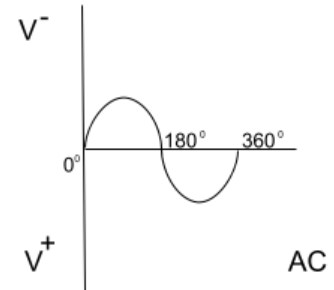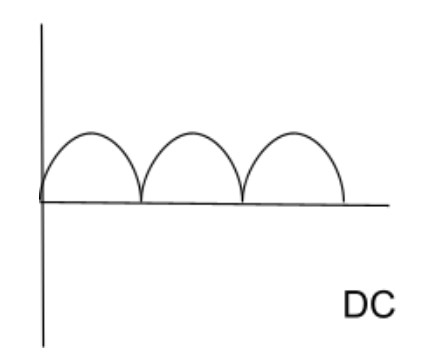# AC to DC Converter Calculator

Enter AC voltage to convert it into DC voltage and vice versa by using this online AC to DC calculator.

Formula:
AC = DC / 0.6360

Volts

Give Us Feedback

AC to DC converter calculator can change the value of alternative current to direct current. It can also work the other way round. It means it can also work as DC to AC calculator.

## What are AC and DC?

Wikipedia defines AC as:

“The electric current which periodically reverses direction and changes its magnitude continuously with time.”Whereas DC is completely the opposite.

“DC is an electric current that has the same direction and magnitude all the time.”## AC to DC conversion formula

DC is equivalent to the average AC value in 90 percent of cases.

`DC = 0.636 × Vp`

`AC = Vp/√2`

Where,

Vp represents the peak voltage.

## How to convert AC into DC?

Example:

What is DC voltage if the peak value of AC is 150 volts?

Solution:

Step 1: Write down the formula.

`DC = 0.636 × Vp`

Step 2: Put the value of peak voltage into the formula.

= 0.636 × 150

DC = 95.4 Volts

### Math Tools